# Chapter 11: Regression Analysis¶

## Example, page-305¶

In :
# Variable declaration

# Calculation
from scipy import *
from pylab import *

l = array([[20,0.18],[60,0.37],[100,0.35],[140,0.78],[180,0.56],[220,0.75],[260,1.18],[300,1.36],[340,1.17],[380,1.65]])
x = l[:,0]
y = l[:,1]
s1 = sum(square(x)) - square(sum(x))/10.0
s2 = sum(x*y) - (sum(x)*sum(y))/10.0
s3 = sum(square(y)) - square(sum(y))/10.0

s3 = round(s3,5)

Slope = s2/s1
Slope = round(Slope,5)

c = sum(y)/10.0 - Slope*sum(x)/10.0
c = round(c,3)

# For x = 190 cm/s
value = c + Slope*190
value = round(value,2)

Sum_of_square = s3 - square(s2)/s1
Sum_of_square = round(Sum_of_square,5)

# Result
print "Evaporation coefficient: ",value,"mm(square)/s"
print "sum of squares: ",Sum_of_square

Evaporation coefficient:  0.8 mm(square)/s
sum of squares:  0.20238


## Example, Page-306¶

In :
# Variable declaration
n = 50
Meanx = 88.34
Meany = 305.58
s1 = 7239.22
s2 = 17840.1
s3 = 66975.2
x = []
for i in range(60,120):
x.append(i)

# Calculation
from scipy import *
from pylab import *
%matplotlib inline

x = array(x)
Slope1 = s2/s1
Slope1 = round(Slope1,3)

c1 = Meany - (s2/s1)*Meanx
c1 = round(c1,2)

Slope2 = s2/s3
Slope2 = round(Slope2,3)

c2 = Meanx - (s2/s3)*Meany

# Result
print "Part-A: ","Height = ",c1,"+",Slope1,"*width"
print "Part-B: ","Height = ",round(-c2/Slope2,2),"+",round(1/Slope2,3),"*width"

plot(x,c1+Slope1*x)
plot(x,-c2/Slope2 + x/Slope2)
legend(['height = 87.88 + 2.464*width', 'height = -26.11 + 3.759*width'])
xlabel("$Width$")
ylabel("$Height$")

Part-A:  Height =  87.88 + 2.464 *width
Part-B:  Height =  -26.1 + 3.759 *width

Out:
<matplotlib.text.Text at 0xa58bf60>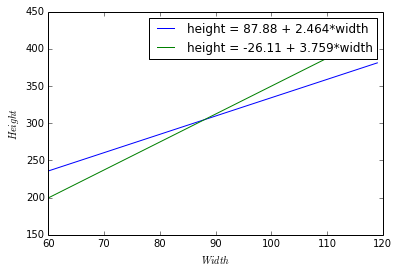## Example, Page-308¶

In :
# Variable declaration

# Calculation
from scipy import *
from pylab import *
import numpy as np

a = np.array([[10,2000], [2000,532000]])
b = np.array([8.35,2175.40])
x = np.linalg.solve(a, b)

# Result
print "a =",round(x,3)
print "b =",round(x,5)

a = 0.069
b = 0.00383


## Example, page-311¶

In :
# Variable declaration

# Calculation
from scipy import *
from pylab import *

l = array([[20,0.18],[60,0.37],[100,0.35],[140,0.78],[180,0.56],[220,0.75],[260,1.18],[300,1.36],[340,1.17],[380,1.65]])
x = l[:,0]
y = l[:,1]

s1 = sum(square(x)) - square(sum(x))/10.0
s2 = sum(x*y) - (sum(x)*sum(y))/10.0
s3 = sum(square(y)) - square(sum(y))/10.0
s3 = round(s3,5)

Slope = s2/s1
Slope = round(Slope,5)

c = sum(y)/10.0 - Slope*sum(x)/10.0
c = round(c,3)

s = (s3 - square(s2)/s1) / 8       # estimation of sigma square
s = round(sqrt(s),3)

# 95% confidence interval
t_thr = 2.306       # at 0.025 and v = 8

y1 = c - ( (t_thr)*s* sqrt(1.0/10 + square(sum(x)/10)/float(s1) ) )
y1 = round(y1,3)
y2 = c + ( (t_thr)*s* sqrt(1.0/10 + square(sum(x)/10)/float(s1) ) )
y2 = round(y2,3)

# Result
print "95% confidence interval: (",y1,",",y2,")"

95% confidence interval: ( -0.164 , 0.302 )


## Example, Page-311¶

In :
# Variable declaration
l = array([[20,0.18],[60,0.37],[100,0.35],[140,0.78],[180,0.56],[220,0.75],[260,1.18],[300,1.36],[340,1.17],[380,1.65]])
alpha = 0.05
beta = 0.0

# Calculation
from scipy import *
from pylab import *

x = l[:,0]
y = l[:,1]
s1 = sum(square(x)) - square(sum(x))/10.0
s2 = sum(x*y) - (sum(x)*sum(y))/10.0
s3 = sum(square(y)) - square(sum(y))/10.0
s3 = round(s3,5)

t_thr = 2.306   # theoritical t-value t(0.025)

Slope = s2/s1
Slope = round(Slope,5)

Sum_of_square = (s3 - square(s2)/s1)/8
Se = sqrt(Sum_of_square)
Se = round(Se,3)

c = sum(y)/10.0 - Slope*sum(x)/10.0
c = round(c,3)

t_prt = ((Slope)/Se)*sqrt(s1)
t_prt = round(t_prt,2)

# Result
print "Practical t-value: ",t_prt
if(t_prt > t_thr):
print "null hypothesis is rejected"
print "Relationship between air velocity & average evaporation cofficient EXISTS"
else:
print "null hypothesis is accepted"
print "No Relationship between air velocity & average evaporation cofficient EXISTS"

Practical t-value:  8.75
null hypothesis is rejected
Relationship between air velocity & average evaporation cofficient EXISTS


## Example, Page-313¶

In :
# Variable declaration
l = array([[20,0.18],[60,0.37],[100,0.35],[140,0.78],[180,0.56],[220,0.75],[260,1.18],[300,1.36],[340,1.17],[380,1.65]])
alpha = 0.05
beta = 0.0
x0 = 190

# Calculation
from scipy import *
from pylab import *

x = l[:,0]
y = l[:,1]
s1 = sum(square(x)) - square(sum(x))/10.0
s2 = sum(x*y) - (sum(x)*sum(y))/10.0
s3 = sum(square(y)) - square(sum(y))/10.0
s3 = round(s3,5)

t_thr = 2.306   # theoritical t-value t(0.025)

Slope = s2/s1

Sum_of_square = (s3 - square(s2)/s1)/8
Se = sqrt(Sum_of_square)
Se = round(Se,3)

c = sum(y)/10.0 - Slope*sum(x)/10.0
c = round(c,3)

y1 = (c+Slope*x0) - (t_thr*Se)*sqrt(1.0/10 + ((x0-mean(x))**2)/float(s1))   # Lower limit
y2 = (0.8) + (t_thr*Se)*sqrt(1.0/10 + ((x0-mean(x))**2)/float(s1))   # Lower limit

y1 = round(y1,3)
y2 = round(y2,2)

# Result
print "95% confidence interval(in gallons): (",y1,",",y2,")"

95% confidence interval(in gallons): ( 0.68 , 0.92 )


## Example, page-315¶

In :
# Variable declaration
l = array([[20,0.18],[60,0.37],[100,0.35],[140,0.78],[180,0.56],[220,0.75],[260,1.18],[300,1.36],[340,1.17],[380,1.65]])
air_vel = 190        # air velocity in cm/s

# Calculation
from scipy import *
from pylab import *

x = l[:,0]
y = l[:,1]

s1 = sum(square(x)) - square(sum(x))/10.0
s2 = sum(x*y) - (sum(x)*sum(y))/10.0
s3 = sum(square(y)) - square(sum(y))/10.0

s3 = round(s3,5)

Slope = s2/s1
Slope = round(Slope,5)

c = sum(y)/10.0 - Slope*sum(x)/10.0
c = round(c,3)

# 95% confidence interval
t_thr = 2.306       # at 0.025 and v = 8

s = (s3 - square(s2)/s1) / 8       # estimation of sigma square
s = round(sqrt(s),3)

# For x = 190 cm/s
value = c + Slope*190
value = round(value,2)

y1 = value - ( (t_thr)*s* sqrt(1 + 1.0/10 + square(air_vel - sum(x)/10.0)/float(s1) ) )
y2 = value + ( (t_thr)*s* sqrt(1 + 1.0/10 + square(air_vel - sum(x)/10.0)/float(s1) ) )
y1 = round(y1,2)
y2 = round(y2,2)

# Result
print "95% confidence interval: (",y1,",",y2,")"

95% confidence interval: ( 0.42 , 1.18 )


## Example, page-315¶

In :
# Variable declaration
l = array([[20,0.18],[60,0.37],[100,0.35],[140,0.78],[180,0.56],[220,0.75],[260,1.18],[300,1.36],[340,1.17],[380,1.65]])
air_vel = 450        # air velocity in cm/s

# Calculation
from scipy import *
from pylab import *

x = l[:,0]
y = l[:,1]

s1 = sum(square(x)) - square(sum(x))/10.0
s2 = sum(x*y) - (sum(x)*sum(y))/10.0
s3 = sum(square(y)) - square(sum(y))/10.0

s3 = round(s3,5)

Slope = s2/s1
Slope = round(Slope,5)

c = sum(y)/10.0 - Slope*sum(x)/10.0
c = round(c,3)

# 95% confidence interval
t_thr = 2.306       # at 0.025 and v = 8

s = (s3 - square(s2)/s1) / 8       # estimation of sigma square
s = round(sqrt(s),3)

# For x = 190 cm/s
y1 = 1.79 - ( (t_thr)*s* sqrt(1 + 1.0/10 + square(air_vel - sum(x)/10.0)/float(s1) ) )
y2 = 1.79 + ( (t_thr)*s* sqrt(1 + 1.0/10 + square(air_vel - sum(x)/10.0)/float(s1) ) )
y1 = round(y1,2)
y2 = round(y2,2)

# Result
print "95% confidence interval(in mm(square)/s): (",y1,",",y2,")"

95% confidence interval(in mm(square)/s): ( 1.33 , 2.25 )


## Example, page-321¶

In :
# Calculation
from scipy import *
from pylab import *
%matplotlib inline

l = array([[1,98.2],[2,91.7],[5,81.3],[10,64.0],[20,36.4],[30,32.6],[40,17.1],[50,11.3]])
x = l[:,0]
y = l[:,1]
logy = log10(y)

x_sum = sum(x)
x_sq_sum = sum(square(x))
logy_sum = sum(logy)
xlogy_sum = sum(x*logy)

s1 = (x_sq_sum) - square(x_sum)/8.0
s2 = (xlogy_sum) - (x_sum)*(logy_sum)/8.0

scatter(x,logy)

title("Logrithmic Graph")
xlabel("x")
ylabel("logy")
c = 2.0002
d = -0.0188
# equation logy = c - d*x

logy = c - d*25

# taking antilog   y = 33.9%

# Result
print "% of high performance tries that will last at least 25000 miles: 33.9%"

% of high performance tries that will last at least 25000 miles: 33.9%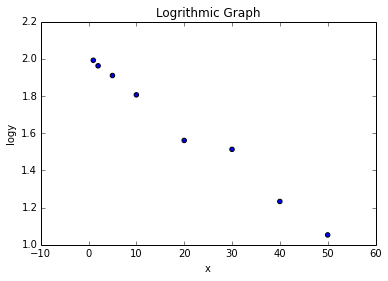## Example, Page-324¶

In :
# Variable Declaration
l = array([[0,12.0],[1,10.5],[2,10.0],[3,8.0],[4,7.0],[5,8.0],[6,7.5],[7,8.5],[8,9.0]])
X = 6.5

# Calculation
from scipy import *
from pylab import *
import numpy as np
%matplotlib inline

x = l[:,0]
y = l[:,1]

x_sum = sum(x)
x_sq_sum = sum(square(x))
x_cube_sum = sum(square(x)*x)
x_four_sum = sum(square(x)*square(x))

y_sum = sum(y)
xy_sum = sum(x*y)
x2y_sum = sum(x*x*y)

a = np.array([[9,x_sum,x_sq_sum], [x_sum,x_sq_sum,x_cube_sum],[x_sq_sum,x_cube_sum,x_four_sum]])
b = np.array([y_sum,xy_sum,x2y_sum])
val = np.linalg.solve(a, b)
val = round(val,1)
val = round(val,2)
val = round(val,3)

scatter(x,y)
title("Scatter plot")
xlabel("x")
ylabel("y")

# Result
print "Least square polynomial: y=",val,val,"*x(1)+",val,"*x(2)"
# for x = 6.5
print "y =",round(val + val*X + val*(X**2),1),"hours"

Least square polynomial: y= 12.2 -1.85 *x(1)+ 0.183 *x(2)
y = 7.9 hours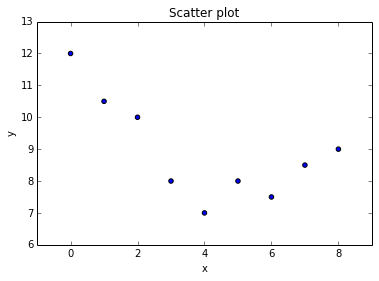## Example, Page-327¶

In :
# Variable declaration
l = array([[41,1,5],[49,2,5],[69,3,5],[65,4,5],[40,1,10],[50,2,10],[58,3,10],
[57,4,10],[31,1,15],[36,2,15],[44,3,15],[57,4,15],[19,1,20],[31,2,20],
[33,3,20],[43,4,20]])
X1 = 2.5
X2 = 12

# Calculation
from scipy import *
from pylab import *
import numpy as np

y = l[:,0]
x1 = l[:,1]
x2 = l[:,2]

x1_sum = sum(x1)
x1_sq_sum = sum(square(x1))
x2_sum = sum(x2)
x2_sq_sum = sum(square(x2))
x1x2_sum = sum(x1*x2)

y_sum = sum(y)
x1y_sum = sum(x1*y)
x2y_sum = sum(x2*y)

a = np.array([[16,x1_sum,x2_sum], [x1_sum,x1_sq_sum,x1x2_sum],[x2_sum,x1x2_sum,x2_sq_sum]])
b = np.array([y_sum,x1y_sum,x2y_sum])
val = np.linalg.solve(a, b)
val = round(val,1)
val = round(val,2)
val = round(val,2)

# Result
print "Estimated regression plane: y=",val,"+",val,"*x1",val,"*x2"
print "y =",round(val + val*X1 + val*X2,1)

Estimated regression plane: y= 46.4 + 7.78 *x1 -1.65 *x2
y = 46.0


## Example, page-336¶

In :
# Variable Declaration

# Calculation
from scipy import *
from pylab import *
%matplotlib inline

l = array([[11.1,10.9],[10.3,14.2],[12.0,13.8],[15.1,21.5],[13.7,13.2],[18.5,21.1],[17.3,16.4],[14.2,19.3],[14.8,17.4],[15.3,19.0]])
x = l[:,0]
y = l[:,1]

scatter(x,y)
title("y Vs x")
xlabel("$x$")
ylabel("$y$")

s1 = sum(square(x)) - square(sum(x))/10.0
s2 = sum(x*y) - (sum(x)*sum(y))/10.0
s3 = sum(square(y)) - square(sum(y))/10.0

# correlation coefficient
r = s2 / sqrt(s1*s3)
r = round(r,3)

# Result
print "correlation coefficient: ",r

correlation coefficient:  0.732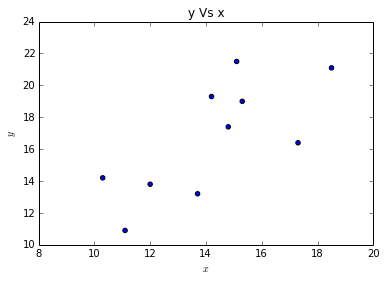## Example, Page-337¶

In :
# Variable Declaration
l = array([[250,19],[290,10],[270,17],[100,11],[300,70],[410,60],[110,18],[130,30],[1100,180]])

# Calculation
from scipy import *
from pylab import *
%matplotlib inline

x = l[:,0]
y = l[:,1]

figure()
scatter(x,y)
title("y Vs x")
xlabel("$x$")
ylabel("$y$")

s1 = sum(square(x)) - square(sum(x))/9.0
s2 = sum(x*y) - (sum(x)*sum(y))/9.0
s3 = sum(square(y)) - square(sum(y))/9.0

r = s2 / sqrt(s1*s3)
r = round(r,3)
print "correlation coefficient: ",r

for i in range(0,9):
for j in range(0,2):
l[i,j] = math.log(l[i,j])

lnx = l[:,0]
lny = l[:,1]

figure()
scatter(lnx,lny)
title("lny Vs lnx")
xlabel("$lnx$")
ylabel("$lny$")

s1 = sum(square(lnx)) - square(sum(lnx))/9.0
s2 = sum(lnx*lny) - (sum(lnx)*sum(lny))/9.0
s3 = sum(square(lny)) - square(sum(lny))/9.0

# correlation coefficient
r = s2 / sqrt(s1*s3)
r = round(r,3)
print "correlation coefficient: ",r

correlation coefficient:  0.942
correlation coefficient:  0.75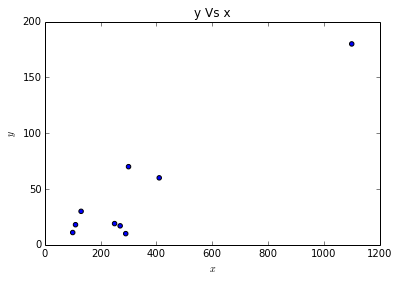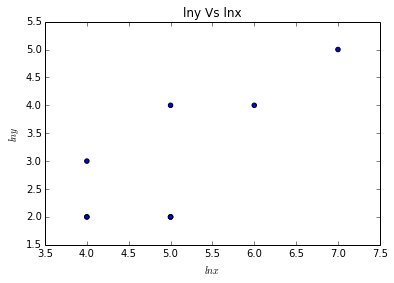## Example, Page-339¶

In :
# Variable Declaration
l = array([[11.1,10.9],[10.3,14.2],[12.0,13.8],[15.1,21.5],[13.7,13.2],[18.5,21.1],[17.3,16.4],[14.2,19.3],[14.8,17.4],[15.3,19.0]])

# Calculation
from scipy import *
from pylab import *

x = l[:,0]
y = l[:,1]

s1 = sum(square(x)) - square(sum(x))/10.0
s2 = sum(x*y) - (sum(x)*sum(y))/10.0
s3 = sum(square(y)) - square(sum(y))/10.0

# correlation coefficient
r = s2 / sqrt(s1*s3)
r = round(r,3)

# Result
print "around",round(100*(r**2),1),"% of the variation among the afternoon times is explained by the corresponding differenced among the morning times."

around 53.6 % of the variation among the afternoon times is explained by the corresponding differenced among the morning times.


## Example, Page-341¶

In :
# Variable declaration
r = 0.732        # correlation coff;
n = 10

# Calculation
from scipy import *
from pylab import *

# 95% confidence interval
Z1 = 0.5*math.log((1+r)/(1-r))       # Z value corresponds to r=0.7
z_prt = sqrt(n-3)*Z1

z_thr = 1.96           # at 0.025

# Result
print "Practical Z-value: ",round(z_prt,2)
if(z_prt > z_thr):
print "null hypothesis must be rejected"
print "Relationship between morning & afternoon times it takes a mechanic to assemble the machinery, EXISTS"
else:
print "null hypothesis is accepted"
print "Relationship between morning & afternoon times it takes a mechanic to assemble the machinery, does not EXIST"

Practical Z-value:  2.47
null hypothesis must be rejected
Relationship between morning & afternoon times it takes a mechanic to assemble the machinery, EXISTS


## Example, page-342¶

In :
# Variable declaration
r = 0.70        # correlation coff;
n = 30          # number of students

# Calculation
from scipy import *
from pylab import *

# 95% confidence interval
Z = 0.5*log((1+r)/(1-r))
Z = 0.5*math.log((1+r)/(1-r))       # Z value corresponds to r=0.7

z_thr = 1.96           # at 0.025

u1 = Z - (z_thr) / sqrt(27)
u2 = Z + (z_thr) / sqrt(27)

y1 = (exp(u1) - exp(-u1)) / (exp(u1) + exp(-u1))
y2 = (exp(u2) - exp(-u2)) / (exp(u2) + exp(-u2))
y1 = round(y1,2)
y2 = round(y2,2)

# Result
print "95% confidence interval for normal population: (",y1,",",y2,")"

95% confidence interval for normal population: ( 0.45 , 0.85 )


## Example, Page-346¶

In :
# Variable declaration
n = 16
X1 = 2.5
X2 = 12

# Calculation
from scipy import *
from pylab import *
import numpy as np
from numpy import matrix

l = array([[41,1,5],[49,2,5],[69,3,5],[65,4,5],[40,1,10],[50,2,10],[58,3,10],
[57,4,10],[31,1,15],[36,2,15],[44,3,15],[57,4,15],[19,1,20],[31,2,20],
[33,3,20],[43,4,20]])
y = l[:,0]
x1 = l[:,1]
x2 = l[:,2]

x1_sum = sum(x1)
x1_sq_sum = sum(square(x1))
x2_sum = sum(x2)
x2_sq_sum = sum(square(x2))
x1x2_sum = sum(x1*x2)

y_sum = sum(y)
x1y_sum = sum(x1*y)
x2y_sum = sum(x2*y)

A = matrix([[n,x1_sum,x2_sum],[x1_sum,x1_sq_sum,x1x2_sum],[x2_sum,x1x2_sum,x2_sq_sum]])
A = A.I

B = matrix([[y_sum],[x1y_sum],[x2y_sum]])

b = A*B

# Result
print "Least square estimates of multiple regression coefficients",b

Least square estimates of multiple regression coefficients [[ 46.4375]
[  7.775 ]
[ -1.655 ]]


## Example, Page-348¶

In :
# Variable declaration
n = 5
k = 1

# Calculation
from scipy import *
from pylab import *
import numpy as np
from numpy import matrix

X = matrix([[1,0],[1,1],[1,2],[1,3],[1,4]])
Y = matrix([,,,,])

XT = X.T

XTX = XT*X
XTXI = XTX.I
XTY = XT*Y

b = XTXI*XTY

Y1 = X*b
MMT = ((Y-Y1).T)*(Y-Y1)
MMT = int(MMT)

Se_square = (1.0/(n-k-1))*MMT

# Result
print "Se Square value: ",Se_square

Se Square value:  2.0


## Example, Page-349¶

In :
# Variable declaration
n = 5
k = 1

# Calculation
from scipy import *
from pylab import *
import numpy as np
from numpy import matrix

X = matrix([[1,0],[1,1],[1,2],[1,3],[1,4]])
Y = matrix([,,,,])

XT = X.T

XTX = XT*X
XTXI = XTX.I
XTY = XT*Y

b = XTXI*XTY

Y1 = X*b
MMT = ((Y-Y1).T)*(Y-Y1)
MMT = int(MMT)

Se_square = (1.0/(n-k-1))*MMT

Final = Se_square*XTXI

# Result
print "var(bo): ",float(Final[0,0])
print "var(b1): ",float(Final[1,1])

var(bo):  1.2
var(b1):  0.2